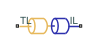# Interface (TL-IL)

Connection between thermal and isothermal networks

Since R2020a

•Libraries:
Simscape / Fluids / Fluid Network Interfaces

## Description

The Interface block is a connector between thermal and isothermal fluid networks. The fluid mass flow rate and pressure are matched between the networks, but fluid properties are not passed between the two networks. To maintain fluid properties between the thermal and isothermal components of your model, connect an Isothermal Liquid Predefined Properties (IL) block with the desired properties to the isothermal network side.

You can connect Thermal Liquid library blocks with Isothermal Liquid library or Hydraulics (Isothermal) library blocks. The temperature set in the isothermal system can either be `Constant` or `Controlled`. In the case of a `Controlled` temperature system, the isothermal network temperature is set by the thermal liquid temperature. In the `Constant` temperature setting, you specify the isothermal network temperature directly.### Thermal Liquid - Isothermal Liquid

When Fluids domain interface is set to ```Thermal Liquid (TL) - Isothermal Liquid (IL)```, the mass flow rate is conserved over the two mass-flow-rate-based networks:

`${\stackrel{˙}{m}}_{TL}+{\stackrel{˙}{m}}_{IL}=0,$`

where:

• $\stackrel{˙}{m}$TL is the mass flow rate at the thermal liquid interface.

• $\stackrel{˙}{m}$IL is the mass flow rate at the isothermal liquid interface.

The fluid absolute pressure is maintained between the thermal, internal, and isothermal sections of the Interface block:

`${p}_{TL}={p}_{IL}.$`

### Thermal Liquid - Hydraulics (Isothermal)

The Hydraulics (Isothermal) Liquid library is based on volumetric flow rate. To maintain flow continuity between both sides of the interface, the volumetric flow rate is multiplied by the fluid density:

`${\stackrel{˙}{m}}_{TL}+{q}_{H}{\rho }_{H}=0,$`

where:

• qH is the Hydraulics (Isothermal) volumetric flow rate.

• ρH is the local Hydraulics (Isothermal) fluid density.

The Hydraulics (Isothermal) Liquid library blocks are constructed in terms of gauge pressures. Pressure is converted to absolute pressure internally to match the thermal network pressure:

`${p}_{H}+{p}_{atm}={p}_{TL}.$`

## Ports

### Conserving

expand all

Entry or exit port to the thermal liquid network.

Entry or exit port to the isothermal liquid network.

#### Dependencies

To enable this port, set Fluids domain interface to ```Thermal Liquid (TL) - Isothermal Liquid (IL)```.

Entry or exit port to the hydraulics (isothermal) liquid network.

#### Dependencies

To enable this port, set Fluids domain interface to ```Thermal Liquid (TL) - Hydraulic (H)```.

## Parameters

expand all

Specifies the connecting library types.

Sets the isothermal network temperature. In the `Constant` setting, you can specify the isothermal network temperature in the Hydraulic temperature parameter. In the `Controlled` setting, the temperature in the isothermal network is set by the thermal network.

Specifies the fluid temperature transmitted from the thermal liquid network to the hydraulic or isothermal liquid network.

#### Dependencies

To enable this parameter, set Temperature specification to `Constant`.

Cross-sectional area of the thermal liquid port.

## Version History

Introduced in R2020a